Ordering

The Ordering usage message is shown below.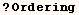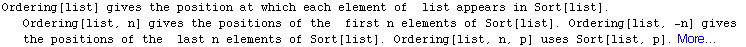The next cell gives us the ordering of a list.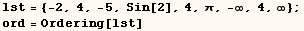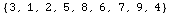The next cell shows how we can take the results of Ordering and get a sorted
list.  When Ordering is given one or two arguments we get the ordering of a
cononical sort which may not be the ordering of a numerically sorted list.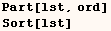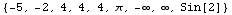Ordering[lst,4]  gives the first four elements of Ordering[lst].  The second
argument of Ordering can be (All) or an Integer meeting the condition
(-Length[list] ≤ n ≤ Length[list]).  A few cells after
this example we use All as a a second argument for Ordering.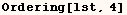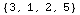The next cell gives the last four elements of Ordering[lst].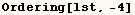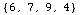The next cell gives the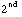through the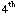elements of  Ordering[lst].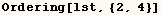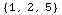The output of the next cell returns with the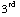element of Ordering[lst], and ends with thefrom the last element of Ordering[lst].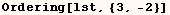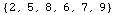The next cell returns every other element of Ordering[lst] starting with the
first element of (lst) and ending with the last element of (lst).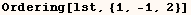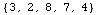As mentioned above Ordering normally gives the ordering of a cononical sort.
We can give Ordering a third argument to get the Ordering of another type of
sort.  In the next cell we get the ordering of a numeric sort.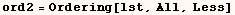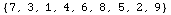The next cell shows how we can take the results of the last Ordering and get
a numerically sorted list.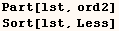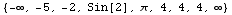In all the examples above Ordering was given a list, but Ordering can work
with expressions having any head as shown in the next cell.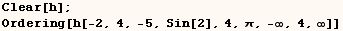Created by Mathematica  (May 16, 2004)

Back to Ted’s Tricks index page